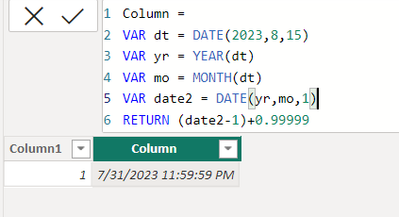cancel
Showing results for
Did you mean:Frequent Visitor

## SQL to DAX

How do I write the following SQL in DAX please

Thank you

1 ACCEPTED SOLUTIONSuper User

``select DATEADD(s,-1,DATEADD(mm,0,DATEADD(mm,DATEDIFF(mm,0,DATEFROMPARTS(2023,8,15)),0)))``

returns

``2023-07-31 23:59:59.000``

You need DAX like this

``````Column =
VAR dt = DATE(2023,8,15)
VAR yr = YEAR(dt)
VAR mo = MONTH(dt)
VAR date2 = DATE(yr,mo,1)
RETURN (date2-1)+0.99999

or

Column =
VAR dt = DATE(2023,8,15)
VAR yr = YEAR(dt)
VAR mo = MONTH(dt)
VAR date2 = DATE(yr,mo,1)
RETURN (date2-1)+CONVERT(TIME(23,59,59),DOUBLE)``````replace GETTODAY() with TODAY() in DAX

Why 0.99999 works here I have no idea,

@AlexisOlson  do you know why it works? Can we achieve that part through a calculation rather than hardcoding

Proud to be a Super User!

New Animated Dashboard: Sales Calendar

5 REPLIES 5Super User

``select DATEADD(s,-1,DATEADD(mm,0,DATEADD(mm,DATEDIFF(mm,0,DATEFROMPARTS(2023,8,15)),0)))``

returns

``2023-07-31 23:59:59.000``

You need DAX like this

``````Column =
VAR dt = DATE(2023,8,15)
VAR yr = YEAR(dt)
VAR mo = MONTH(dt)
VAR date2 = DATE(yr,mo,1)
RETURN (date2-1)+0.99999

or

Column =
VAR dt = DATE(2023,8,15)
VAR yr = YEAR(dt)
VAR mo = MONTH(dt)
VAR date2 = DATE(yr,mo,1)
RETURN (date2-1)+CONVERT(TIME(23,59,59),DOUBLE)``````replace GETTODAY() with TODAY() in DAX

Why 0.99999 works here I have no idea,

@AlexisOlson  do you know why it works? Can we achieve that part through a calculation rather than hardcoding

Proud to be a Super User!

New Animated Dashboard: Sales CalendarSuper User

@smpa01 You're subtracting a day and then adding back almost a whole day.

(1-0.99999) days = 0.000001 days = 0.864 seconds.

So, essentially, you're taking midnight on the first of the month and subtracting roughly one second.

Since this is in the Power Query forum, I'd recommend

``Date.StartOfMonth(DateTime.LocalNow()) - #duration(0,0,0,1)``

In DAX, I'd recommend

``EOMONTH ( TODAY(), -1 ) + TIME ( 23, 59, 59 )``Frequent Visitor

Thank you I'll have a play with this.Super User

Hi @SuzieKidd - Could you please explain what you are trying to achieve with this formula?

Please note that the DAX DATEADD function does not support seconds, minutes and hours like its SQL cousin.  You may consider using the add duration functionality in Power Query, or add a DAX functions that convert datetime to seconds (a bit like UNIX Timestamp).Frequent Visitor

Thank you